# MCQs on Electrical And Electronics Measurements

##### Page 9 of 19. Go to page 1 2 3 4 5 6 7 8 9 10 11 12 13 14 15 16 17 18 19
01․ Potentiometer method of DC voltage measurement is more accurate than direct measurement using a voltmeter because
it loads the circuit to maximum extent.
it uses centre zero galvanometer instead of voltmeter.
it does not load the circuit at all.

Since the circuit is not loaded, the accurate value of DC voltage is measured.

02․ Assertion (A): A hot wire ammeter has a cramped scale. Reason (R): The heat is proportional to square of current.
Both A and R are true and R is correct explanation of A.
Both A and R are true and R is not correct explanation of A.
A is ture and R is false.
A is false and R is ture.

In hot wire ammeter, heating is proportional to square of rms value of operating current, Hence scale is cramped.

03․ The items in Group-I represent the various types of measurement to be made with a reasonable accuracy using a suitable bridge. The items in Group-II represent the various bridges available for this purpose. Select the correct choice of the item in Group-II for the corresponding item in Group-I from the following Group I:
• P. Resistance in milli-ohm range.
• Q. Low values of capacitance.
• R. Comparison of resistance which are nearly equal.
• S. Inductance of a coil with a large time constant.
Group-II:
1. Wheatstone bridge.
2. Kelvin double bridge.
3. Schering bridge.
4. Weins bridge
5. Hays bridge
6. Carey-foster bridge
P = 2, Q = 3, R = 6, S = 5.
P = 2, Q = 6, R = 4, S = 5.
P = 2, Q = 3, R = 5, S = 4.
P = 1, Q = 3, R = 2, S = 6.

Kelvin double bridge is used for measuring low values of resistance (P = 2). Low values of capacitance is precisely measured by Schering bridge (Q = 3). Comparison of resistance which are nearly equal is done by Carey-foster bridge (R = 6). Inductance of a coil with large time constant or high quality factor is measured by Hays bridge (S = 5).

04․ A Manganin swap resistance is connected in series with a moving coil ammeter consisting of a milli ammeter and a suitable shunt in order to
Minimize the effect of temperature variation.
Obtaine large deflecting torque.
Reduce the size of the meter.
Minimize the effect of stray magnetic fields.

In PMMC instruements, as temperature increases the coil resistance increases. Swap resistance are connected in series with the moving coil to provide temperature compensation.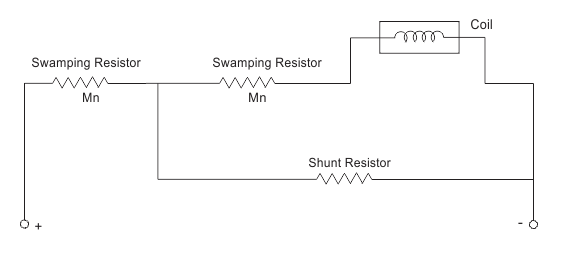Swamping resistors is made of magnin which has a zero temperature coefficient.

05․ A 50 Hz bar primary CT has a secondary with 500 turns. The secondary supplies 5 A current into a purely resistive burden of 1Ω. The magnetizing ampere-turn is 200. The phase angle between the primary and secondary current is
4.6o.
85.4o.
94.6o.
175.4o.

For small values of phase angle,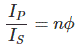Where, n is turns ratio and φ is phase angle. Magnetizing ampere turns = 200. So primary current Ip = 200 × 1 = 200 amp. Turn ratio n = 500. Secondary current Is = 5 amp.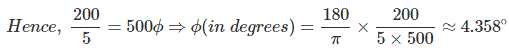06․ The core flux in the CT of above question no.6, under the given operating conditions is
0.
450 mWb.
225 mWb.
1000 mWb.

Voltage appeared at secondary winding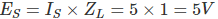Voltage induced is given by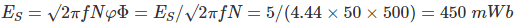07․ A moving coil of a meter has 100 turns, and a length and depth of 10 mm and 20 mm respectively. It is postioned in a uniform radial flux density of 200 mT. The coil carries a current of 50 mA. The torque on the coil is
200 Nm.
100 Nm.
2 Nm.
1 Nm.

The torque on the coil is given by τ = NIBA. Where N = no. of turn, I = current, B = magnetic field density, A = Area.

08․ A single phase load is connected between R and Y terminals of a 415 V, symmetrical, 3-phase, 4 wire system with phase sequence RYB. A wattmeter is connected in the system as shown in figure. The power factor of the load is 0.8 lagging. The wattmeter will read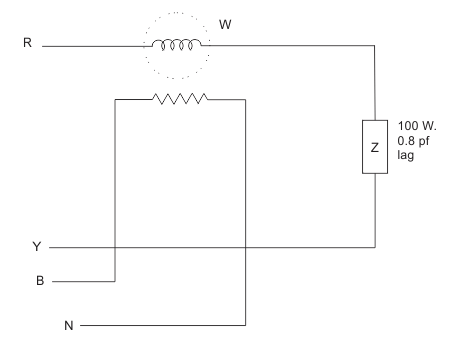- 795 W.
- 597 W.
+ 597 W.
+ 795 W.

In the figure, VRY = 415∠30° VBN = (415/√3∠120°) current in current coil,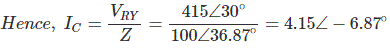Reading of wattmeter, P = 994.3(cos 126.87°) = 994.3(-0.60) = - 597 W.

09․ A moving iron ammeter produces a full scale torque of 240 μNm with a deflection of 120° at a current of 10 A. The rate of change of self induction (μH/radian) of the instrument at full scale is